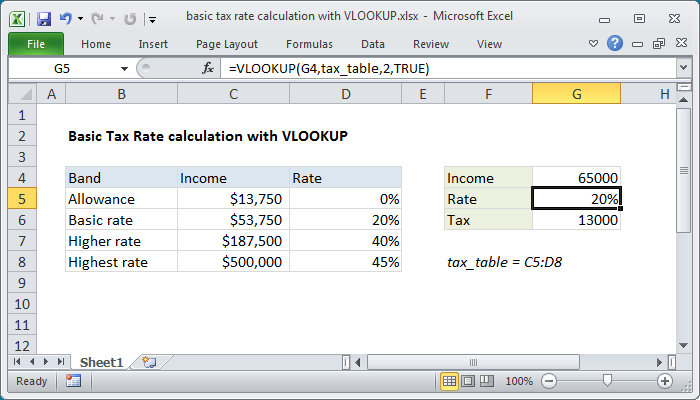# How To Using Excel VBA for Tax Rates

Oct 16, 2020 • edited Oct 19, 2020Below we will look at a program in Excel VBA that calculates the tax on an income. The following tax rates apply to individuals who are residents of Australia.

Taxable incomeTax on this income
0 - \$6,000Nil
\$6,001 - \$35,00015c for each \$1 over \$6,000
\$35,001 - \$80,000\$4,350 plus 30c for each \$1 over \$35,000
\$80,001 - \$180,000\$17,850 plus 38c for each \$1 over \$80,000
\$180,001 and over\$55,850 plus 45c for each \$1 over \$180,000

Situation:1. First, we declare two double variables. One double variable we call income, and one double variable we call tax.

Dim income As Double

Dim tax As Double

2. We initialize the variable income with the value of cell A2 and round it.

income = Round(Range(“A2”).Value)

3. We place the rounded value into cell A2 again.

Range(“A2”).Value = income

4. We use the Select Case statement to calculate the tax on an income. Excel VBA uses income to test each subsequent Case statement to see if the code under the Case statement should be executed.

Select Case income

Case Is >= 180001

tax = 55850 + 0.45 * (income - 180000)

Case Is >= 80001

tax = 17850 + 0.38 * (income - 80000)

Case Is >= 35001

tax = 4350 + 0.3 * (income - 35000)

Case Is >= 6001

tax = 0.15 * (income - 6000)

Case Else

tax = 0

End Select

Example: if income is 37000, tax equals 4350 + 0.3 * (37000-35000) = 4350 + 600 = \$4950

5. We write the value of the variable tax to cell B2.

Range(“B2”).Value = tax

6. Place this code in a command button and test it.

Result:#Tutorial#How To#VBA#If Then Statement

How To Use Userform with Multiple Pages In Excel

Variables and Variable Scope In Excel VBA